CBSE Class 12 Sample Paper for 2018 Boards

Class 12
Solutions of Sample Papers and Past Year Papers - for Class 12 Boards

### 2 e sin⁡bx - 1 /bx, if x > 0

This is a question of CBSE Sample Paper - Class 12 - 2017/18.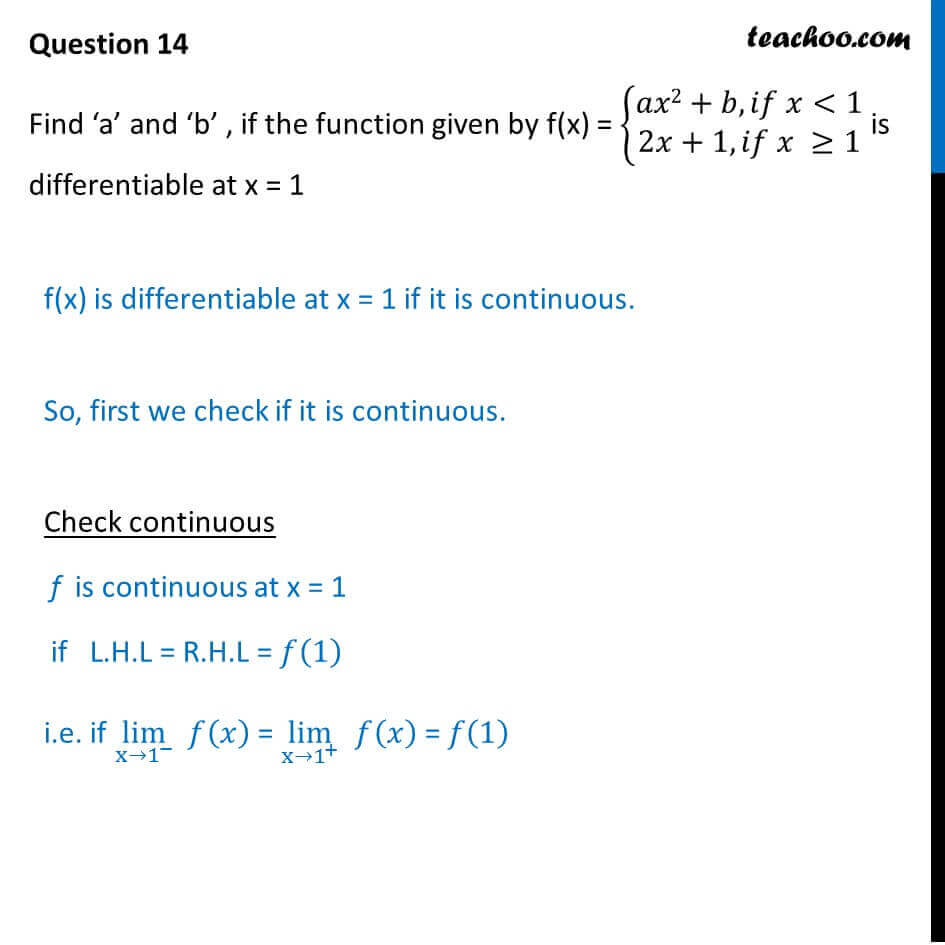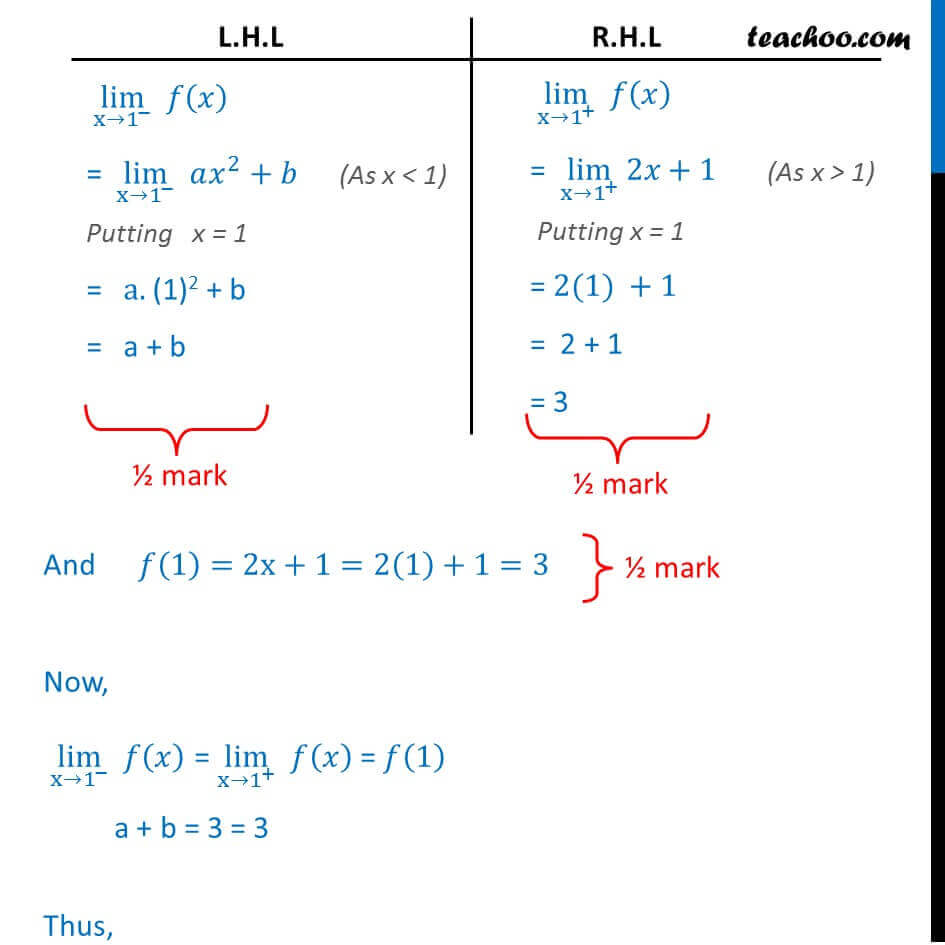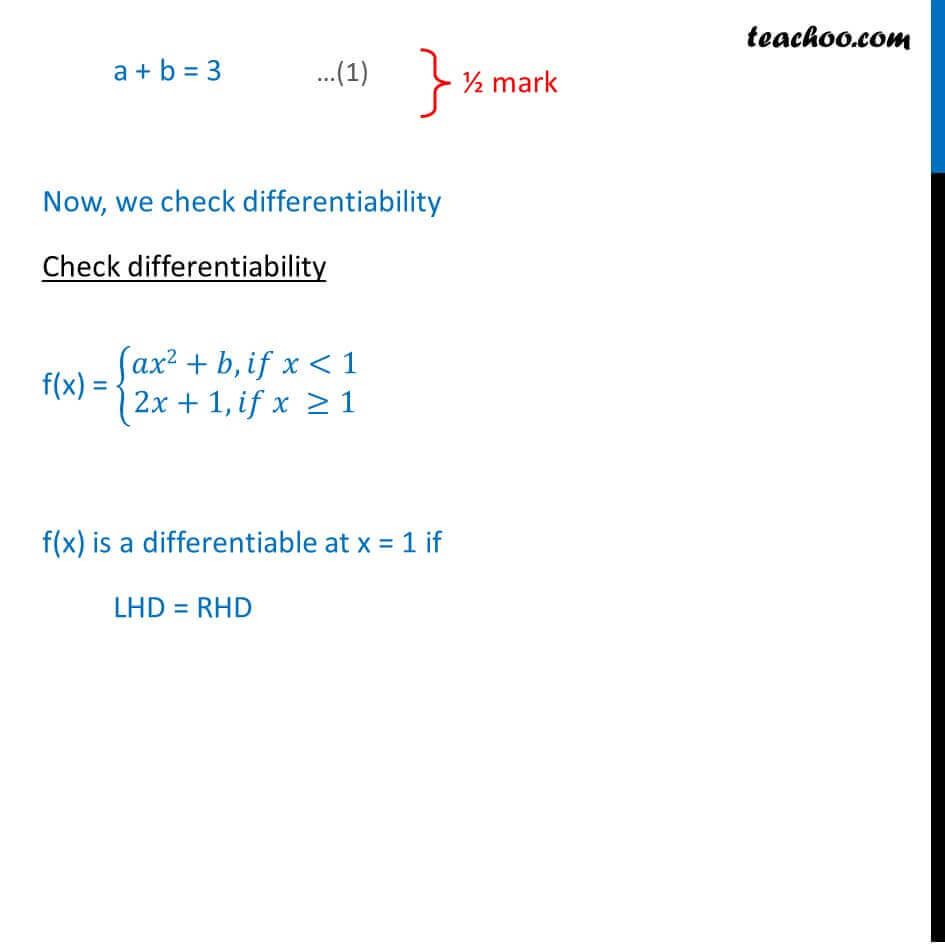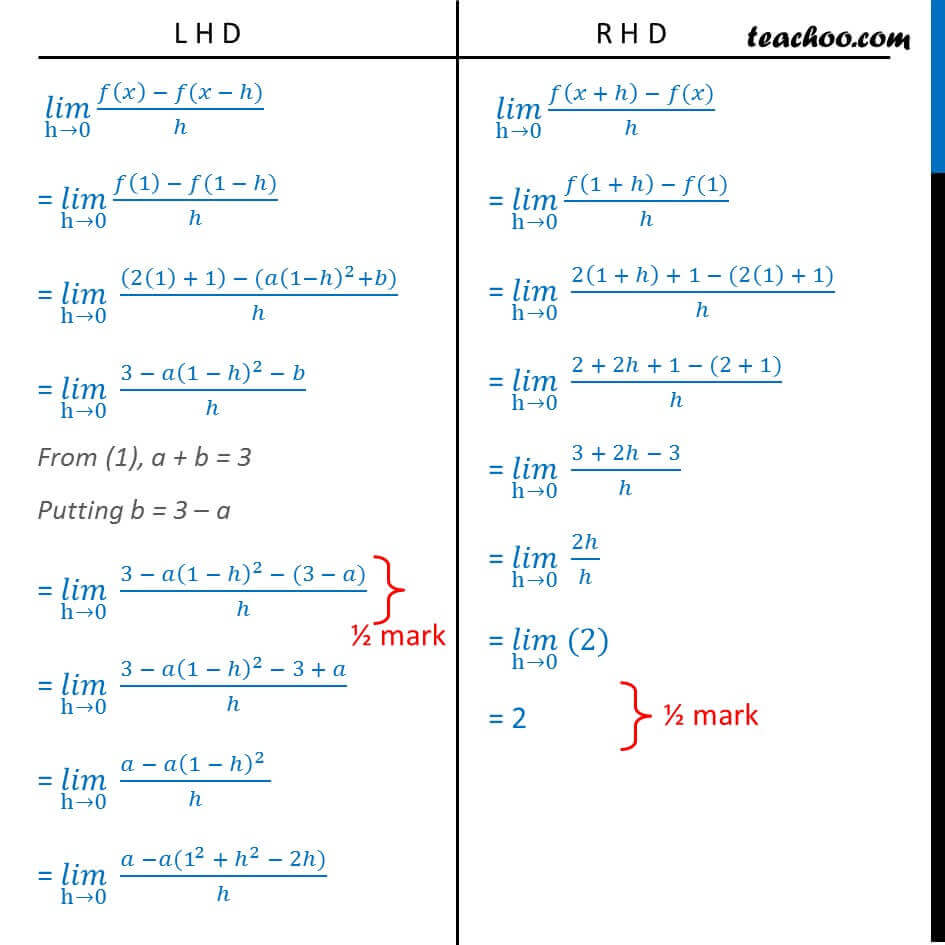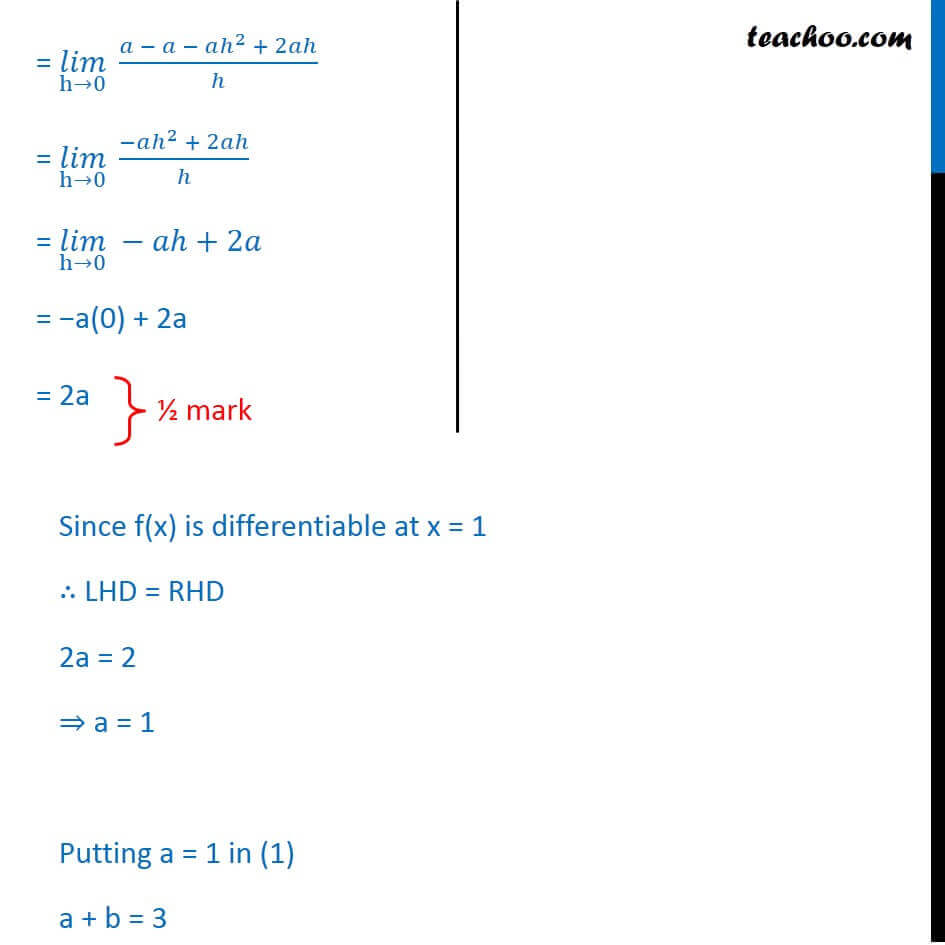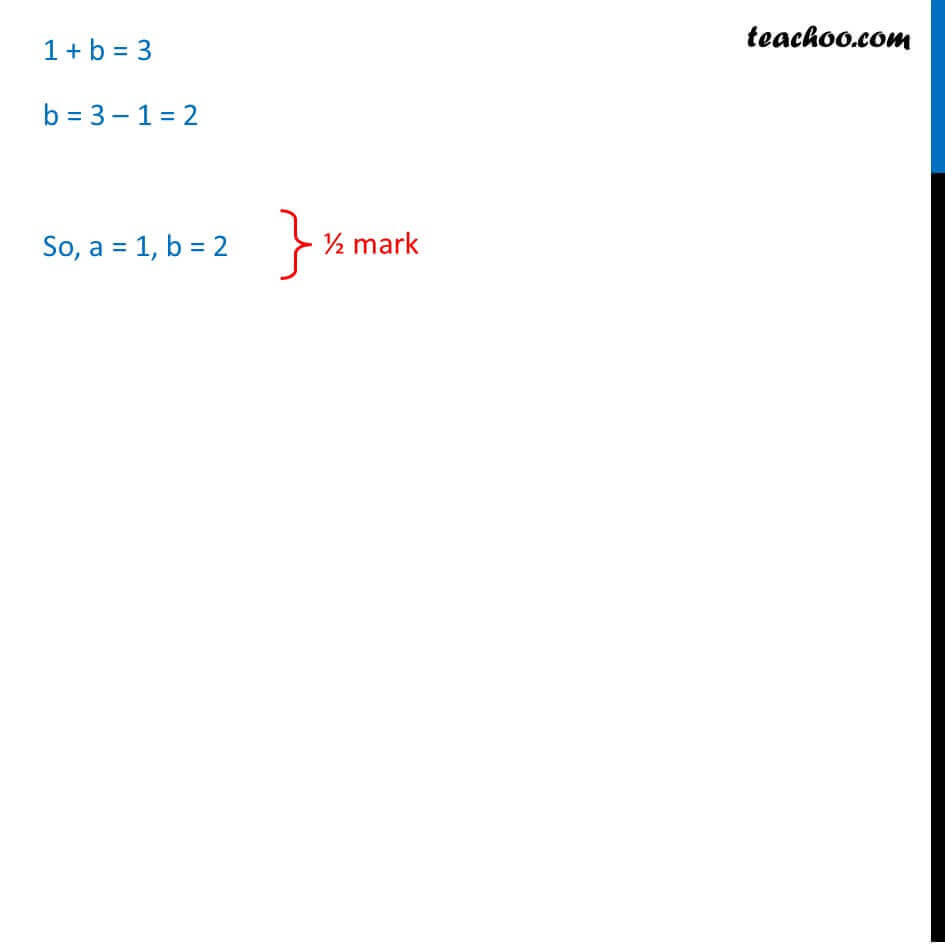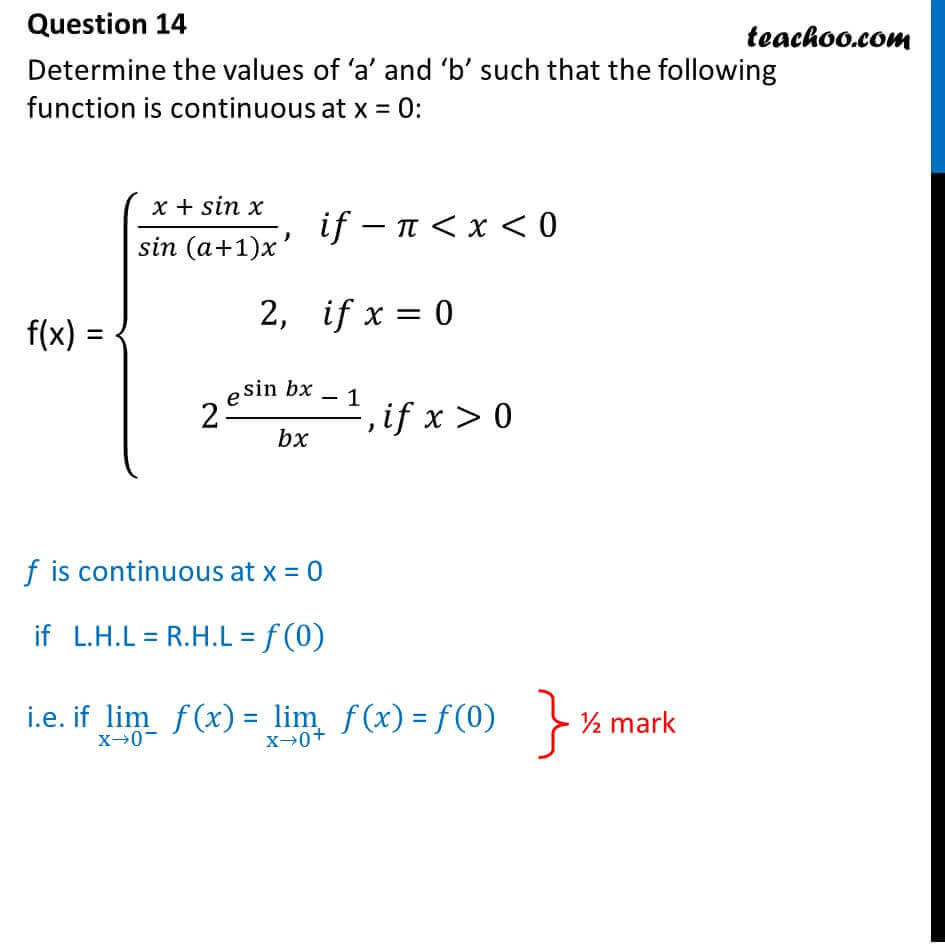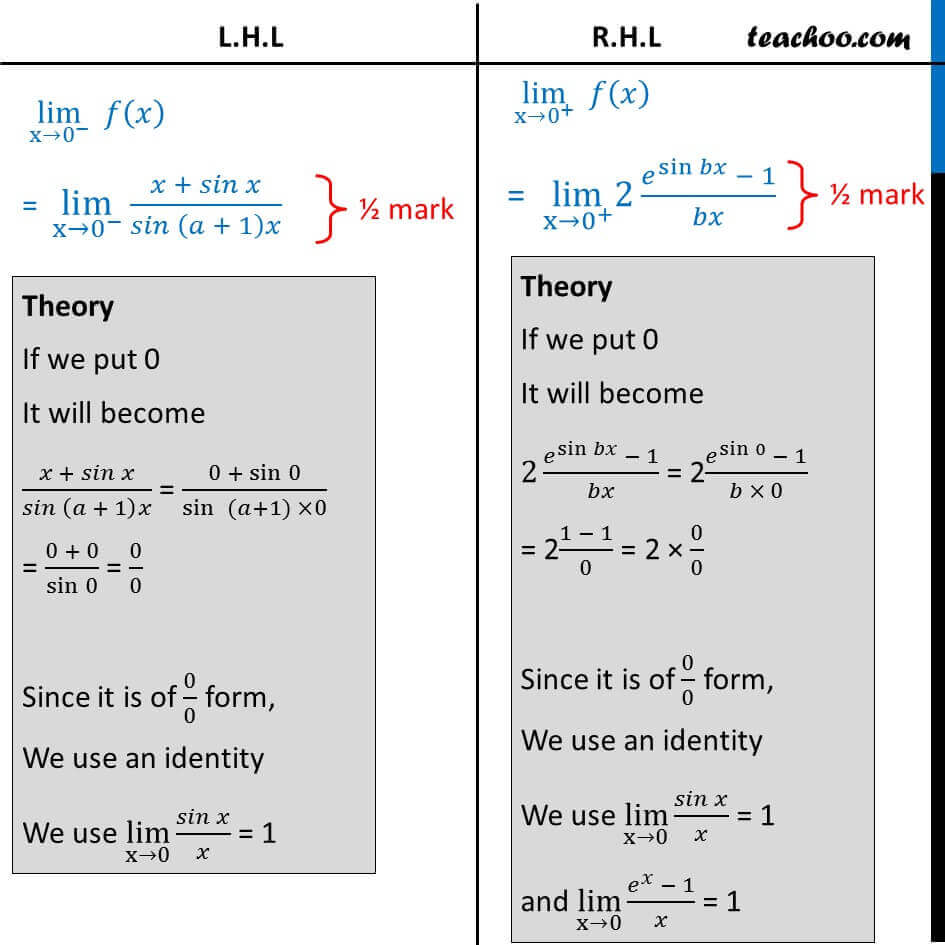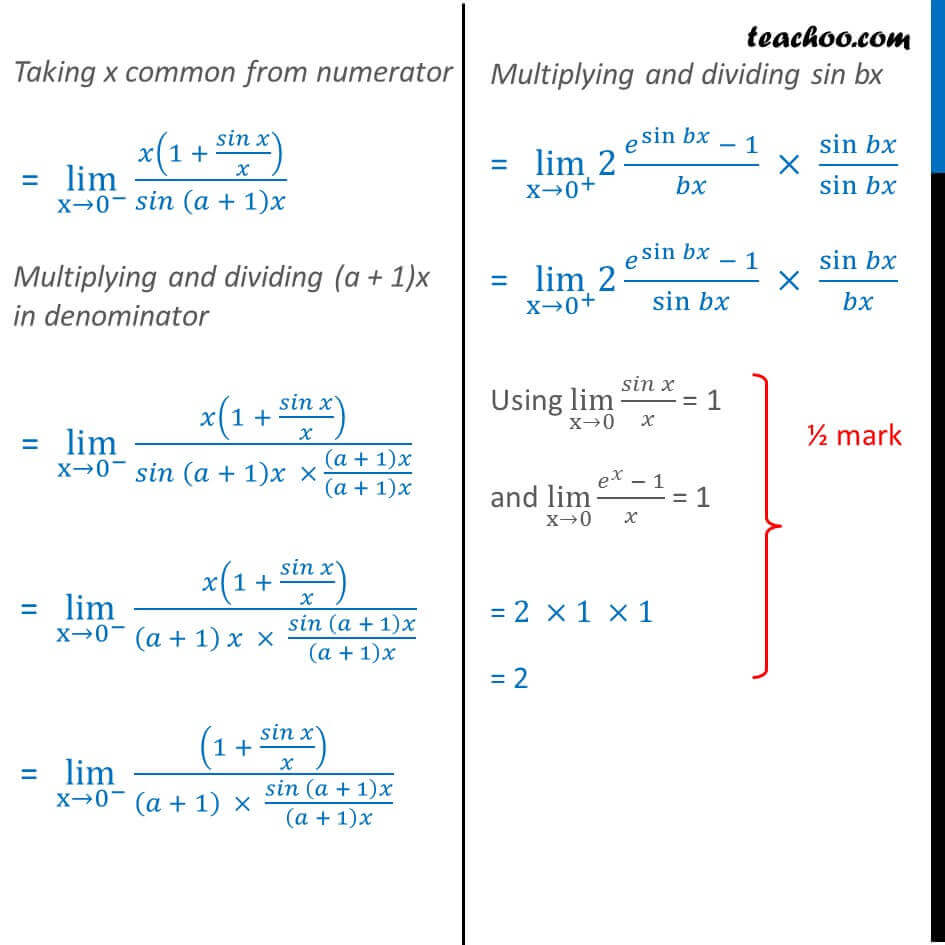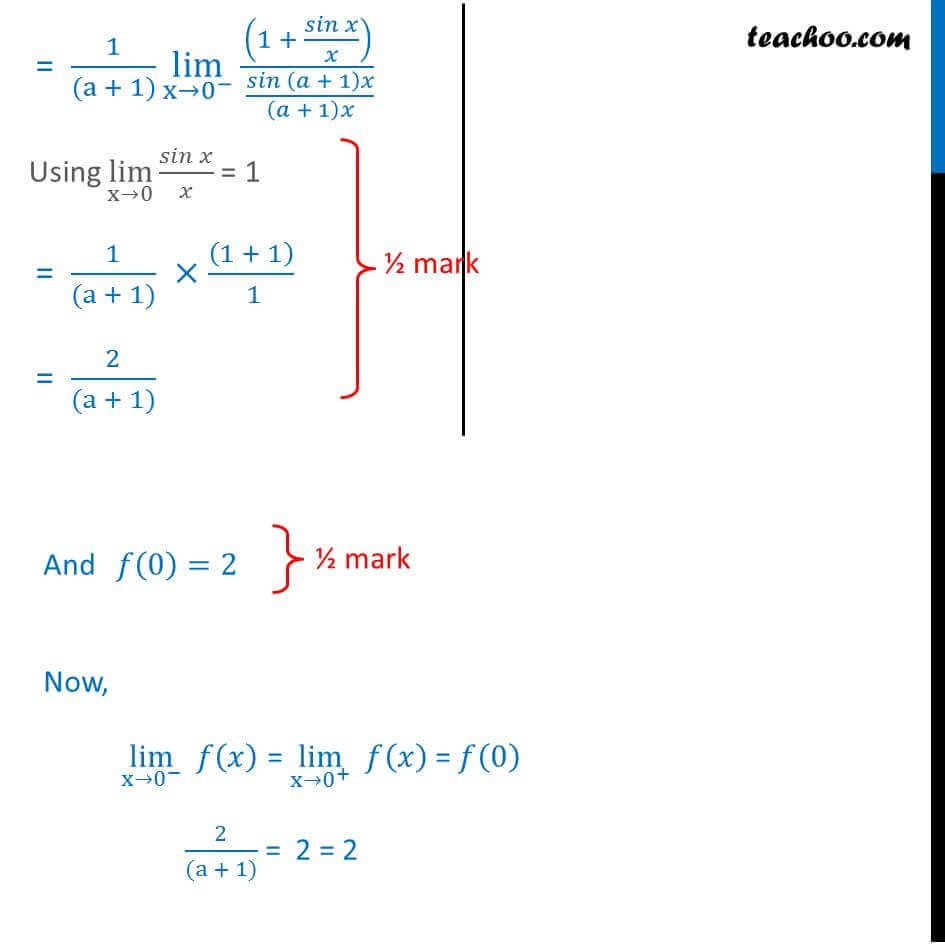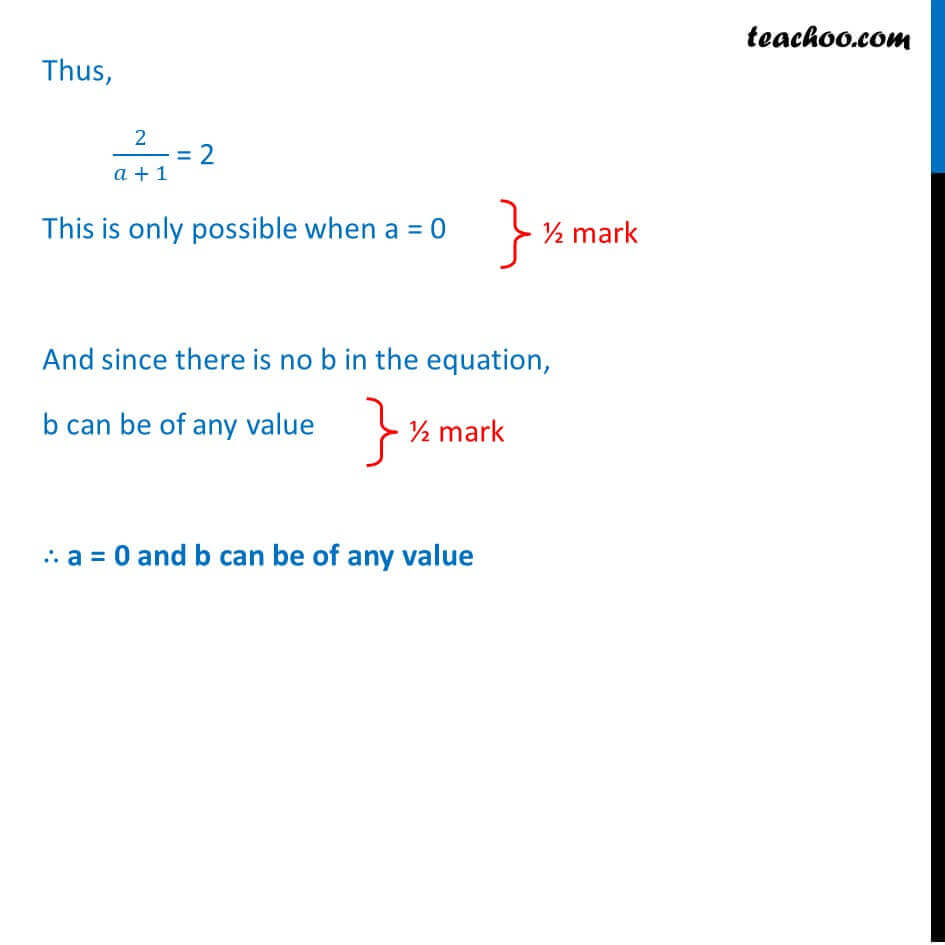Learn in your speed, with individual attention - Teachoo Maths 1-on-1 Class

### Transcript

Question 14 Find a and b , at if the function given by f(x) = { ( 2+ , <1@2 +1, 1) is differentiable x = 1f(x) is differentiable at x = 1 if it is continuous. So, first we check if it is continuous. Check continuous f is continuous at x = 1 if L.H.L = R.H.L = (1) i.e. if lim (x 1^ ) ( ) = lim (x 1^+ ) ( ) = (1) lim (x 1^ ) ( ) = lim (x 1^ ) ^2+ Putting x = 1 = a. (1)2 + b = a + b lim (x 1^+ ) ( ) = lim (x 1^+ ) 2 +1 Putting x = 1 = 2(1) +1 = 2 + 1 = 3 lim (x 1^+ ) ( ) = lim (x 1^+ ) 2 +1 Putting x = 1 = 2(1) +1 = 2 + 1 = 3 And (1)=2x+1=2(1)+1=3 Now, lim (x 1^ ) ( ) = lim (x 1^+ ) ( ) = (1) a + b = 3 = 3 Thus, a + b = 3 Now, we check differentiability Check differentiability f(x) = { ( 2+ , <1@2 +1, 1) f(x) is a differentiable at x = 1 if LHD = RHD ( ) (h 0) ( ( ) ( ))/ = ( ) (h 0) ( (1) (1 ))/ = ( ) (h 0) ((2(1) + 1) ( (1 )^2+ ))/ = ( ) (h 0) (3 (1 )^2 )/ From (1), a + b = 3 Putting b = 3 a = ( ) (h 0) (3 (1 )^2 (3 ))/ = ( ) (h 0) (3 (1 )^2 3 + )/ = ( ) (h 0) ( (1 )^2 )/ = ( ) (h 0) ( (1^2 + ^2 2 ))/ ( ) (h 0) ( ( + ) ( ))/ = ( ) (h 0) ( (1 + ) (1))/ = ( ) (h 0) (2(1 + ) + 1 (2(1) + 1))/ = ( ) (h 0) (2 + 2 + 1 (2 + 1))/ = ( ) (h 0) (3 + 2 3)/ = ( ) (h 0) 2 / = ( ) (h 0) (2) = 2= ( ) (h 0) ( + ^2 + 2 )/ = ( ) (h 0) ( ^2 + 2 )/ = ( ) (h 0) +2 = a(0) + 2a = 2a = ( ) (h 0) ( + ^2 + 2 )/ = ( ) (h 0) ( ^2 + 2 )/ = ( ) (h 0) +2 = a(0) + 2a = 2a = ( ) (h 0) ( + ^2 + 2 )/ = ( ) (h 0) ( ^2 + 2 )/ = ( ) (h 0) +2 = a(0) + 2a = 2a = ( ) (h 0) ( + ^2 + 2 )/ = ( ) (h 0) ( ^2 + 2 )/ = ( ) (h 0) +2 = a(0) + 2a = 2a Since f(x) is differentiable at x = 1 LHD = RHD 2a = 2 a = 1 Putting a = 1 in (1) a + b = 3 1 + b = 3 b = 3 1 = 2 So, a = 1, b = 2 Question 14 Determine the values of a and b such that the following function is continuous at x = 0: f(x) = { (( + )/( ( +1) ), < <0 @ @2, =0@ @2 ( ^sin 1)/ , >0@ ) f is continuous at x = 0 if L.H.L = R.H.L = (0) i.e. if lim (x 0^ ) ( ) = lim (x 0^+ ) ( ) = (0) lim (x 0^ ) ( ) = lim (x 0^ ) ( + )/( ( + 1) ) Theory If we put 0 It will become ( + )/( ( + 1) ) = (0 + sin 0)/sin ( +1) 0 = (0 + 0)/sin 0 = 0/0 Since it is of 0/0 form, We use an identity We use lim (x 0) ( )/ = 1 lim (x 0^+ ) ( ) = lim (x 0^+ ) 2 ( ^sin 1)/ Theory If we put 0 It will become 2 ( ^sin 1)/ = 2( ^sin 0 1)/( 0) = 2(1 1)/0 = 2 0/0 Since it is of 0/0 form, We use an identity We use lim (x 0) ( )/ = 1 and lim (x 0) ( ^ 1)/ = 1 Taking x common from numerator = lim (x 0^ ) (1 + ( )/ )/( ( + 1) ) Multiplying and dividing (a + 1)x in denominator = lim (x 0^ ) (1 + ( )/ )/( ( + 1) ( + 1) /( + 1) ) = lim (x 0^ ) (1 + ( )/ )/(( + 1) ( ( + 1) )/( + 1) ) = lim (x 0^ ) ((1 + ( )/ ))/(( + 1) ( ( + 1) )/( + 1) )Multiplying and dividing sin bx = lim (x 0^+ ) 2 ( ^sin 1)/ sin /sin = lim (x 0^+ ) 2 ( ^sin 1)/sin sin / Using lim (x 0) ( )/ = 1 and lim (x 0) ( ^ 1)/ = 1 = 2 1 1 = 2 = 1/((a + 1)) lim (x 0^ ) ((1 + ( )/ ))/( ( ( + 1) )/( + 1) ) Using lim (x 0) ( )/ = 1 = 1/((a + 1)) ((1 + 1))/( 1) = 2/((a + 1)) And (0)=2 Now, lim (x 0^ ) ( ) = lim (x 0^+ ) ( ) = (0) 2/((a + 1)) = 2 = 2 Thus, 2/( + 1) = 2 This is only possible when a = 0 And since there is no b in the equation, b can be of any value a = 0 and b can be of any value